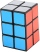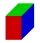# Cuboid + surface area - math problems

#### Number of problems found: 99

• A butterA butter cube with an edge 6.5 cm long is packed in a package with dimensions a = 28 cm, b = 15 cm. Calculate how many cm2 the package is larger than the surface of the cube.
• Empty aquariumHow much does an empty aquarium weigh with dimensions: length = 40 cm, width = 30 cm, height = 20 cm, if 1 dm2 of glass weighs 300 g? Calculate its weight in kilograms.
• Regular square prismThe volume of a regular square prism is 192 cm3. The size of its base edge and the body height is 1: 3. Calculate the surface of the prism.
• Cloth / textileWe have cloth measure 16 square meters. How many 20 cm by 20 cm by 8 cm bags you can make? Assume bag is a cuboid without one top base.
• Roll of wallpaperAn art student uses a roll of wallpaper to decorate two gift boxes. The student will use 3 1/3 yards of paper for one box and 5/6 yard of paper for the other box. The paper must be cut into pieces that are 1/6 yard long. How many pieces will the student c
• Cuboid - ratioFind the volume of a block whose dimensions are in the ratio 2: 3: 4 and the surface is 117 dm2.
• Cuboid edgesThe lengths of the cuboid edges are in the ratio 2: 3: 4. Find their length if you know that the surface of the cuboid is 468 m2.
• FlowerpotThe block-shaped flowerpot has external dimensions: length 1.25 m, width 10 cm, and height 11 cm. The thickness of the boards from which it is made is 0.8 cm. How many liters of soil is needed to fill it 1 cm below the top edge? What surface do we have to
• Insulate houseThe property owner wants to insulate his house. The house has these dimensions 12, and 12 m is 15 m high. The windows have 6 with dimensions 170 and 150 cm. Entrance doors are 250 and 170 cm in size. How many square meters of polystyrene does he need?
• Cuboid diagonalsThe cuboid has dimensions of 15, 20 and 40 cm. Calculate its volume and surface, the length of the body diagonal and the lengths of all three wall diagonals.
• Surface of the cuboidFind the surface of the cuboid if its edges have the dimensions a, 2/3a, 2a.
• Edge cFind the edge c of cuboid if an edge a = 20 mm, b = 30 mm and surface area S = 8000 mm2.
• Milk cartonsHow much paper do we need for 12 tetra packs with dimensions 6 cm, 11 cm, and 20 cm? Will 1 liter of milk fit in the box?
• Four prismsQuestion No. 1: The prism has the dimensions a = 2.5 cm, b = 100 mm, c = 12 cm. What is its volume? a) 3000 cm2 b) 300 cm2 c) 3000 cm3 d) 300 cm3 Question No.2: The prism base is a rhombus with a side length of 30 cm and a height of 27 cm. The height of t
• Flower boxesHow many m2 of 10mm thick boards are needed to make 12 flower boxes? The dimensions of the box are 180,150 and 1500 mm.
• Cuboid edgesCalculate the volume and surface of a cuboid whose edge lengths are in the ratio 2: 3: 4 and the longest edge measures 10cm.
• Cuboid to cubeA cuboid with dimensions of 9 cm, 6 cm, and 4 cm has the same volume as a cube. Calculate the surface of this cube.
• Surface and volumeFind the surface and volume of a cuboid whose dimensions are 1 m, 50 cm, and 6 dm.
• Volume and surface areaFind the volume and surface of a wooden block with dimensions: a = 8 cm, b = 10 cm, c = 16 cm.
• Fire tank1428 hl of water is filled in a block-shaped fire tank with the edges of the base 12 m and 7 m. Calculate the content of water-wetted areas.

Do you have an exciting math question or word problem that you can't solve? Ask a question or post a math problem, and we can try to solve it.

We will send a solution to your e-mail address. Solved examples are also published here. Please enter the e-mail correctly and check whether you don't have a full mailbox.

Cuboid Problems. Examples for the calculation of the surface area of ​​the solid object .